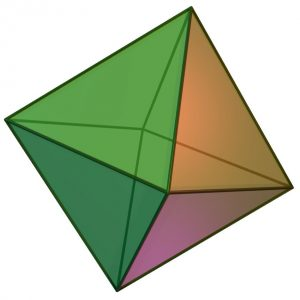# Program to calculate volume of Octahedron

Given the side of the Octahedron then calculate the volume of Octahedron.

Examples:

```Input : 3
Output : 12.7279

Input : 7
Output : 161.692
```

## Recommended: Please try your approach on {IDE} first, before moving on to the solution.

A regular Octahedron has eight faces,twelve edges and six vertices. It has eight triangles with edges of equal length and effectively two square pyramids meeting at their bases.Image Source : Wikimedia

Properties of Octahedron:
Number of faces: 8
Number of edges: 12
Number of vertices: 6

Volume = √2/3 × a3 where a is the side of Octahedron

## CPP

 `// CPP Program to calculate  ` `// volume of Octahedron ` `#include ` `using` `namespace` `std; ` ` `  `// utility Function ` `double` `vol_of_octahedron(``double` `side) ` `{ ` `    ``return` `((side*side*side)*(``sqrt``(2)/3)); ` `} ` ` `  `// Driver Function ` `int` `main() ` `{ ` `    ``double` `side = 3; ` `    ``cout << ``"Volume of octahedron ="` `         ``<< vol_of_octahedron(side) ` `         ``<< endl; ` `} `

## Java

 `// Java Program to calculate  ` `// volume of Octahedron ` ` `  `import` `java.io.*; ` `class` `GFG  ` `{ ` `    ``public` `static` `void` `main (String[] args)  ` `      ``{ ` `          ``// Driver Function ` `         ``double` `side = ``3``; ` `        ``System.out.print(``"Volume of octahedron = "``); ` `        ``System.out.println(vol_of_octahedron(side)); ` ` `  `      ``} ` ` `  `    ``// utility Function ` `    ``static` `double` `vol_of_octahedron(``double` `side) ` `      ``{ ` `         ``return` `((side*side*side)*(Math.sqrt(``2``)/``3``)); ` `      ``} ` `} ` ` `  `// This code is contributed ` `// by Azkia Anam. `

## Python3

 `# Python3 Program to calculate  ` `# volume of Octahedron ` ` `  `import` `math ` ` `  `# utility Function ` `def` `vol_of_octahedron(side): ` `    ``return` `((side``*``side``*``side)``*``(math.sqrt(``2``)``/``3``)) ` ` `  `# Driver Function ` `side ``=` `3` `print``(``"Volume of octahedron ="``, ` `      ``round``(vol_of_octahedron(side),``4``)) ` ` `  `# This code is contributed ` `# by Azkia Anam. `

## C#

 `// C# Program to calculate  ` `// volume of Octahedron ` `using` `System; ` ` `  `class` `GFG  ` `{ ` `    ``public` `static` `void` `Main ()  ` `    ``{ ` `        ``// Driver Function ` `        ``double` `side = 3; ` `        ``Console.Write(``"Volume of octahedron = "``); ` `        ``Console.WriteLine(vol_of_octahedron(side)); ` ` `  `    ``} ` ` `  `    ``// utility Function ` `    ``static` `double` `vol_of_octahedron(``double` `side) ` `    ``{ ` `        ``return` `((side*side*side)*(Math.Sqrt(2)/3)); ` `    ``} ` `} ` ` `  `// This code is contributed ` `// by vt_m. `

## PHP

 ` `

Output:

``` Volume of octahedron = 12.7279
```

Attention reader! Don’t stop learning now. Get hold of all the important DSA concepts with the DSA Self Paced Course at a student-friendly price and become industry ready.

My Personal Notes arrow_drop_upCheck out this Author's contributed articles.

If you like GeeksforGeeks and would like to contribute, you can also write an article using contribute.geeksforgeeks.org or mail your article to contribute@geeksforgeeks.org. See your article appearing on the GeeksforGeeks main page and help other Geeks.

Please Improve this article if you find anything incorrect by clicking on the "Improve Article" button below.

Improved By : vt_m